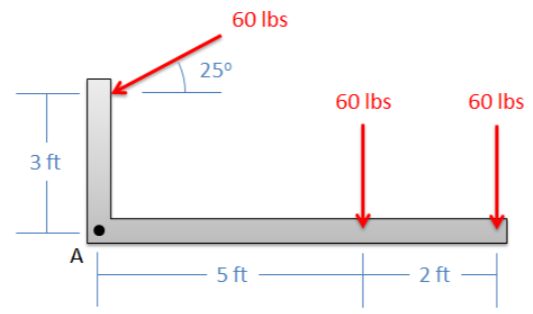# 4.6: Chapter 4 Homework Problems

$$\newcommand{\vecs}{\overset { \rightharpoonup} {\mathbf{#1}} }$$ $$\newcommand{\vecd}{\overset{-\!-\!\rightharpoonup}{\vphantom{a}\smash {#1}}}$$$$\newcommand{\id}{\mathrm{id}}$$ $$\newcommand{\Span}{\mathrm{span}}$$ $$\newcommand{\kernel}{\mathrm{null}\,}$$ $$\newcommand{\range}{\mathrm{range}\,}$$ $$\newcommand{\RealPart}{\mathrm{Re}}$$ $$\newcommand{\ImaginaryPart}{\mathrm{Im}}$$ $$\newcommand{\Argument}{\mathrm{Arg}}$$ $$\newcommand{\norm}{\| #1 \|}$$ $$\newcommand{\inner}{\langle #1, #2 \rangle}$$ $$\newcommand{\Span}{\mathrm{span}}$$ $$\newcommand{\id}{\mathrm{id}}$$ $$\newcommand{\Span}{\mathrm{span}}$$ $$\newcommand{\kernel}{\mathrm{null}\,}$$ $$\newcommand{\range}{\mathrm{range}\,}$$ $$\newcommand{\RealPart}{\mathrm{Re}}$$ $$\newcommand{\ImaginaryPart}{\mathrm{Im}}$$ $$\newcommand{\Argument}{\mathrm{Arg}}$$ $$\newcommand{\norm}{\| #1 \|}$$ $$\newcommand{\inner}{\langle #1, #2 \rangle}$$ $$\newcommand{\Span}{\mathrm{span}}$$$$\newcommand{\AA}{\unicode[.8,0]{x212B}}$$

Exercise $$\PageIndex{1}$$

Determine if the two systems below are statically equivalent.Figure $$\PageIndex{1}$$: part 1 of the problem diagram for Exercise $$\PageIndex{1}$$. A horizontal bar held off the ground by two supports experiences two point forces at different points along its length.Figure $$\PageIndex{2}$$: part 2 of the problem diagram for Exercise $$\PageIndex{1}$$. A horizontal bar held off the ground by two supports experiences a single point force partway along its length.
Solution

No, they are not equivalent.

Exercise $$\PageIndex{2}$$

Determine if the set of forces in A is statically equivalent to the set of forces and moments in B.Figure $$\PageIndex{3}$$: problem diagram for Exercise $$\PageIndex{2}$$. Two uniform crosses of equal dimensions experience a set of forces (system A, left) and a set of forces and moments (system B, right).
Solution

No, they are not equivalent.

Exercise $$\PageIndex{3}$$

Resolve the force shown below into a force and a couple acting at point A. Draw this force and couple on a diagram of the L-shaped beam.Figure $$\PageIndex{4}$$: problem diagram for Exercise $$\PageIndex{3}$$. An L-shaped beam is held parallel to the ground by a pin joint attached at one end (point A), with a force applied to the other free end of the L shape.
Solution

$$F_A = 150 \, N$$ to the left

$$M_A = 450 \, Nm$$

Exercise $$\PageIndex{4}$$

Find the equivalent force couple system acting at point A for the setup shown below. Draw this force and couple on a diagram of the L-shaped beam.Figure $$\PageIndex{5}$$: problem diagram for Exercise $$\PageIndex{4}$$. An L-shaped beam, whose arms intersect at the point A, experiences several forces acting at different points along the arms.
Solution

$$F_A = 155.2 \, lbs, \, 69.5$$° below the negative $$x$$-axis

$$M_A = -556.9 \, ft \, lbs$$

Exercise $$\PageIndex{5}$$

A helicopter is hovering with the wind force, the force from the tail rotor, and the moment due to drag shown below. Determine the equivalent force couple system at point C. Draw the final force and moment on a new diagram of the helicopter.Figure $$\PageIndex{6}$$: problem diagram for Exercise $$\PageIndex{5}$$. A hovering helicopter with point C at the central hub of its main rotor experiences a force applied at C, a moment about C, and a force applied at a distance from C.
Solution

$$F_{eq} = 858.01 \, N$$ acting at $$16.6$$° below the negative $$x$$-axis

$$M_{eq} = -250 \, N \, m$$

Exercise $$\PageIndex{6}$$

Determine the equivalent point load (magnitude and location) for the distributed force shown below, using integration.Figure $$\PageIndex{7}$$: problem diagram for Exercise $$\PageIndex{6}$$. A horizontal bar attached to a wall at one end experiences a distributed force, which varies linearly, over part of its length.
Solution

$$F_{eq} = 105 \, N$$

$$x_{eq} = 3.29 \, m$$ (measured from wall)

Exercise $$\PageIndex{7}$$

Determine the equivalent point load (magnitude and location) for the distributed force shown below, using integration.Figure $$\PageIndex{8}$$: problem diagram for Exercise $$\PageIndex{7}$$. A horizontal bar attached to a wall experiences a distributed force over its length, with magnitude varying linearly according to a piecewise force function.
Solution

$$F_{eq} = 5400 \, lbs$$

$$x_{eq} = 8 \, ft$$ (measured from wall)

Exercise $$\PageIndex{8}$$

Use the method of composite parts to determine the magnitude and location of the equivalent point load for the distributed force shown below.Figure $$\PageIndex{9}$$: problem diagram for Exercise $$\PageIndex{8}$$. A horizontal bar attached to a wall experiences a distributed force over its length, with magnitude varying linearly according to a piecewise force function.
Solution

$$F_{eq} = 12 \, kN$$

$$x_{eq} = 2.79 \, m$$ (measured from wall)

This page titled 4.6: Chapter 4 Homework Problems is shared under a CC BY-SA 4.0 license and was authored, remixed, and/or curated by Jacob Moore & Contributors (Mechanics Map) via source content that was edited to the style and standards of the LibreTexts platform; a detailed edit history is available upon request.